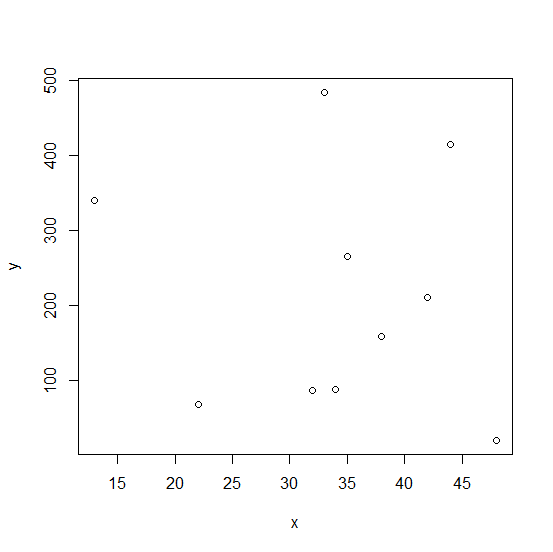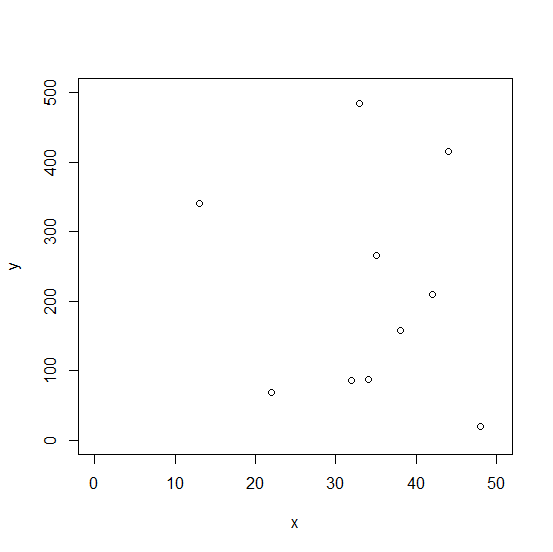# How to change the starting and ending points of axes labels using plot function in R?

R ProgrammingServer Side ProgrammingProgramming

When we create a plot using plot function, the axes labels are automatically created based on the values of the variables that is being plotted. It is possible to set a limit to the labels for both the axes, X-axis and Y-axis and we can do this by using xlim and ylim options. For example, if we have the variable limits from 0 to 50 for variable that is going to be plotted on X-axis then it can be done as xlim = c(0,50).

## Example

set.seed(99)
x <-sample(1:50,10)
y <-sample(1:500,10)

Creating a plot between x and y −

plot(x,y)Creating a plot between x and y by specifying the axes labels −

## Example

plot(x,y,xlim=c(0,50),ylim=c(0,500))

## Output